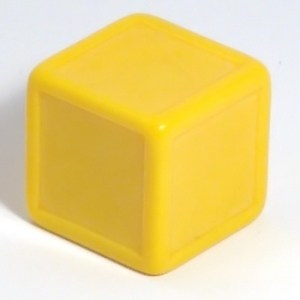# Dice numbering puzzle

1,056.4K Views

James is having 2 dices.
Dice 1- is a normal dice numbered 1 to 6.
Dice 2- is also having 6 faces but with no numbers written on them .
He needs to mark numbers on that 2nd dice so that after throwing both dices simultaneously , all the numbers from 1 to 12 will have equal probability to occur.

How can he do it? and what will be the probability?attach the cubes with a face on another some way. now it is a single cuboidal block . now throwing the block will reveal a number on top of marked dice.
label the unmarked dice with 12-number appeared on dice. repeat. the most distinct opposite faces ,and nearest faces of the dice follow the same rule.

1) To get 1, we require 0 in the other die.
2) Put 0 in 3 faces of the other die
3) To get 7,8,..,12 put 6 in the other 3 faces of the other die.
4) The equal probability of getting each number is  (1*3)/36 = 1/12.

We can mark all the sides of the second sides with 0,0,0,0,0,6,
We have to get the numbers from 1 to 12 to be generated as sum of the no.s appearing on the dice.

By doing this , we get that:
1 -> (1,0)
2 -> (2,0)
3 -> (3,0)
4 -> (4,0)
5 -> (5,0)
6 -> (6,0)
7 -> (1,6)
8 -> (2,6)
9 -> (3,6)
10-> (4,6)
11-> (5,6)
12 -> (6,6)

Every no. has an equal probability of appearance as sum of the no. on the dice.

Probability:

Total combinations possible:

6C1 * 2C1 = 12

Probabilty= 1/12

Hope it helps!!probability of getting 1, 2, 3, 4, 5, 6 = (1*5)/36 = 5/36
probability of getting 7, 8, 9, 10, 11, 12 = (1*1)/36 = 1/36
To avoid this unequal probability only, I have suggested to number 3 faces with 0 and 3 faces with 6!

on 27th May 2016.

7,8,9,10,11,12 on second dice.if 9 reverse is not considered as sixWe need to generate sum from 1 to 12
and probability of sum is asked……

on 4th April 2016.Does it is specified.it says 1 to 12 to occur not 1 to 12 after adding up.remove that down vote as it was not critically obvious

on 6th April 2016.the numbers can’t be marked  randomly.

on 19th April 2016.Probability will same if it is not about sum.even marked randomly.see carefully.question is expectng something else and is something else .i am  just saying that .not meant to attack your ego.but

on 19th April 2016.